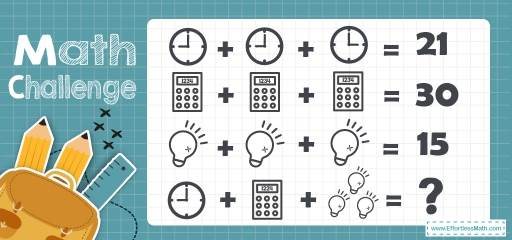# Other Topics Puzzle – Math Challenge 101

This is another perfect math puzzle that can be solved without even using a pen and paper! Paying attention to the details is the key to solve this fascinating Math puzzle!### The Absolute Best Book to challenge your Smart Student!

First, notice that the clocks show different times. The first two clocks show 9 and the third one shows 3. Then: $$9+9+3=21$$

The three calculators sum up to 30. So, each one represents number 10.

The third equation, each lamp represents number 5. $$(5+5+5=15)$$

Now, let’s solve the last equation. The clock represents number 9. This calculator is different from the calculators in the second equation. The numbers in the previous calculators are 1, 2, 3, and 4. We know that the calculators represent number 10. So, $$1+2+3+4=10$$

The calculator on the last equation had numbers 1, 2, 2, and 4. So, the calculator represents number 9: $$1+2+2+4=9$$

Now, notice that the lamps on the last equation are different from the lamp in the third equation. That lamp has 5 bars on it, but these lamps have 4 bars on them. The lamp on the third equation represents number 15. So, 5 bars on the lamp represent 15. Then, each of the lamps with 4 bars represent $$12 \ (4×3=12)$$.

Now, let’s solve the last equation.

$$9+9+12=30$$

### What people say about "Other Topics Puzzle – Math Challenge 101 - Effortless Math: We Help Students Learn to LOVE Mathematics"?

No one replied yet.

X
30% OFF

Limited time only!

Save Over 30%

SAVE $5 It was$16.99 now it is \$11.99